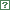Experimental Hall B# CLAS Physics Database

## Search form for the data related to the CLAS physics

You are not logged in. Login.

Select reaction:Select kinematics range:Q2min, [GeV]2:Q2max, [GeV]2:Wmin, [GeV]:Wmax, [GeV]:xmin:xmax:Eγmin, [GeV]:Eγmax, [GeV]:Select observables:
 any σ dσ/dΩ σ_L σ_T σ_L/σ_T σ_TT σ_TL dσ/dt dσ/dt′ σ_LT′ dσ_L/dt dσ_T/dt R_ρ F1 F2 FL g1 g2 A_LU A1p A_UL A_LL dσ/dM(π−,p) dσ/dM(π+,p) dσ/dM(π+,π−) dσ/d(−cos(θ(π−))) dσ/d(−cos(θ(π+))) dσ/d(−cos(θ(p))) dσ/dφ(π−) dσ/dφ(π+) dσ/dφ(p) dσ/dψ(π−) dσ/dψ(π+) dσ/dψ(p) moments of F2 moments of g1 d2σ/dcos(θ_π)dφ_π Abt(θ_π, φ_π) Ab(θ_π, φ_π) At(θ_π, φ_π) σ(θ, φ) Y(+) Y(−) dσ/dP_rel_pn dσ/dP_rel_pp dσ/dP_tot_pn dσ/dP_tot_pp R A_1+ηA_2 g_1^d Γ_1^d dσ/dφ R(4He/3He) R(12C/3He) R(56Fe/3He) dσ/dcos(θK+) F_2 * P(P_t) F_2 * P(P_s) F_2(x*,Q2) r r_00^04 Re r_10^04 r_1-1^04 r_00^1 r_11^1 Re r_10^1 r_1-1^1 Im r_10^2 Im r_1-1^2 r_00^5 r_11^5 Re r_10^5 r_1-1^5 Im r_10^6 Im r_1-1^6 D coeff A_1 g_1/F_1 A_{RC} F_{RC} R_model C_x C_z A_π+ B_π+ C_π+ A_π- B_π- C_π- A_p B_p C_p dσ/(dQ^2 dP_n) dσ/(dQ^2 dP_n dΩ_n) dσ/dcos(θK*) W(cos_θ) W(φ) dσ/dcos(θΞ(1530)−) dσ/dcos(θΞ(1320)−) dσ/dcos(θK+) dσ/dM(K+ K+) dσ/dM(Ξ− K+) dσ/dΩ_T+ε·dσ/dΩ_L Asym_LT Asym_TT dY/dM(e+, e−) dY/dMρ(e+, e−) A_et A_t A R(4He/12C) R(15N/12C) P′ P′ R_GMn GMn_reduced GMn_{NDE} P′_x P′_z Y_00 Y_10 Y_11 Y_20 Y_21 Y_22 Y_30 Y_31 Y_32 Y_33 Y_40 Y_41 Y_42 Y_43 Y_44 d2σ_s/dt dMππ d2σ_p/dt dMππ d2σ_p-/dt dMππ d2σ_p0/dt dMππ d2σ_p+/dt dMππ d2σ_d/dt dMππ d2σ_d-/dt dMππ d2σ_d0/dt dMππ d2σ_d+/dt dMππ d2σ_f/dt dMππ d2σ_f-/dt dMππ d2σ_f0/dt dMππ d2σ_f+/dt dMππ ρ0_0,0 ρ0_1,0 ρ0_1,-1 ρs0_0,0 ρs0_1,0 dσ/dcos(θ) Γ_1^p_meas Γ_1^p_extrp Γ_1^p_tot Γ_1^d_meas Γ_1^d_extrp Γ_1^d_tot γ_0^p_meas γ_0^p_extrp γ_0^p_tot d5σ/dx dQ2 dz dp_T^2 dφ P_Λ s7dσ/dt P_Σ Rm(12C/2H) Rm(56Fe/2H) Rm(208Pb/2H) F2n/F2p A1 A_UL^sin2φ A_LU^sin(φ_h) b σ_T + εσ_L dσ/dcos(θ_HS^π+) T_A(^2H,ω) T_A(^2H,φ) T_A(^{12}C,ω) T_A(^{12}C,φ) d4σ/dQ2 dxb dt dφ d2σ/dcos(θ_K)dφ_K dσ/dM σ_U σ_LTp (d^4σ^+/dQ^2 dxb dt dφ) − (d^4σ^−/dQ2 dxb dt dφ) γ_0 Γ_1 Γ_3 Γ_5 Σ P T Ox Oz A_UL^sinϕ A_LL^const A_LL^cosϕ EAdditional search criteria:Text table options
 Show results as a plain text tableShow average value for W, Q2 rangesAdd W and Q2 columns How to save text data
[] Search Results Table CompositionMember of CLAS Collaboration are encouraged to send available data to the CLAS Physics Database.

You can use our data editing interface (preferred) to enter your structured data or submit your data in arbitrary format using this submission form.

If you have any questions, suggestions or comments, please, feel free to contact developers: